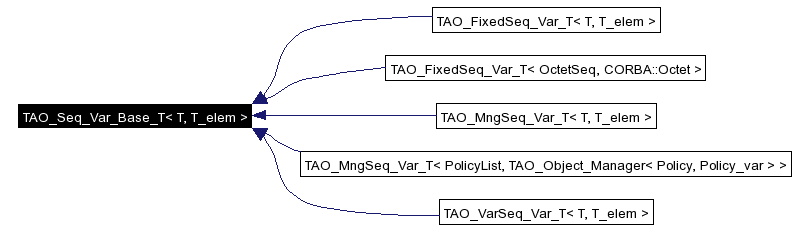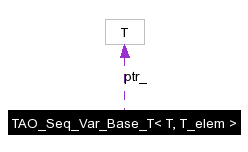# TAO_Seq_Var_Base_T< T, T_elem > Class Template Reference

Parametrized implementation of _var base class for sequences. More...

`#include <Seq_Var_T.h>`

Inheritance diagram for TAO_Seq_Var_Base_T< T, T_elem >:[legend]
Collaboration diagram for TAO_Seq_Var_Base_T< T, T_elem >:[legend]
List of all members.

## Public Types

typedef const T & _in_type
typedef T & _inout_type
typedef T *& _out_type
typedef T * _retn_type

## Public Member Functions

TAO_Seq_Var_Base_T (void)
TAO_Seq_Var_Base_T (T *)
TAO_Seq_Var_Base_T (const TAO_Seq_Var_Base_T< T, T_elem > &)
~TAO_Seq_Var_Base_T (void)
T * operator-> (void)
const T * operator-> (void) const
operator const T & () const
operator T & ()
operator T & () const
_in_type in (void) const
_inout_type inout (void)
_out_type out (void)
_retn_type _retn (void)
_retn_type ptr (void) const

T * ptr_

## Detailed Description

### template<typename T, typename T_elem> class TAO_Seq_Var_Base_T< T, T_elem >

Parametrized implementation of _var base class for sequences.

## Member Typedef Documentation

 template typedef const T& TAO_Seq_Var_Base_T< T, T_elem >::_in_type

 template typedef T& TAO_Seq_Var_Base_T< T, T_elem >::_inout_type

 template typedef T*& TAO_Seq_Var_Base_T< T, T_elem >::_out_type

 template typedef T* TAO_Seq_Var_Base_T< T, T_elem >::_retn_type

## Constructor & Destructor Documentation

 template ACE_INLINE TAO_Seq_Var_Base_T< T, T_elem >::TAO_Seq_Var_Base_T ( void )

 template ACE_INLINE TAO_Seq_Var_Base_T< T, T_elem >::TAO_Seq_Var_Base_T ( T * )

 template TAO_Seq_Var_Base_T< T, T_elem >::TAO_Seq_Var_Base_T ( const TAO_Seq_Var_Base_T< T, T_elem > & )

 template ACE_INLINE TAO_Seq_Var_Base_T< T, T_elem >::~TAO_Seq_Var_Base_T ( void )

## Member Function Documentation

 template ACE_INLINE T * TAO_Seq_Var_Base_T< T, T_elem >::_retn ( void )

 template ACE_INLINE const T & TAO_Seq_Var_Base_T< T, T_elem >::in ( void ) const

 template ACE_INLINE T & TAO_Seq_Var_Base_T< T, T_elem >::inout ( void )

 template ACE_INLINE TAO_Seq_Var_Base_T< T, T_elem >::operator const T & ( ) const

 template ACE_INLINE TAO_Seq_Var_Base_T< T, T_elem >::operator T & ( ) const

 template ACE_INLINE TAO_Seq_Var_Base_T< T, T_elem >::operator T & ( )

 template ACE_INLINE const T * TAO_Seq_Var_Base_T< T, T_elem >::operator-> ( void ) const

 template ACE_INLINE T * TAO_Seq_Var_Base_T< T, T_elem >::operator-> ( void )

 template ACE_INLINE T *& TAO_Seq_Var_Base_T< T, T_elem >::out ( void )

 template ACE_INLINE T * TAO_Seq_Var_Base_T< T, T_elem >::ptr ( void ) const

## Member Data Documentation

 template T* TAO_Seq_Var_Base_T< T, T_elem >::ptr_` [protected]`

The documentation for this class was generated from the following files:
Generated on Sun May 15 13:10:36 2005 for TAO by1.3.9.1